# Specific Resistance of the Material of a Wire using Meter Bridge and Post Office Box

## Problems from IIT JEE

Problem (IIT JEE 2014):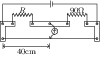During an experiment with a metre bridge, the galvanometer shows a null point when the jockey is pressed at 40.0 cm using a standard resistance of $90\; \Omega$, as shown in the figure. The least count of the scale used in the metre bridge is 1 mm. The unknown resistance is,

1. $60\pm0.15\;\Omega$
2. $135\pm0.56\;\Omega$
3. $60\pm0.25\;\Omega$
4. $135\pm0.23\;\Omega$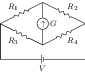Solution: Let $\lambda$ be resistance per unit length (in ohm/cm) of the potentiometer wire. Total length of the wire is 100 cm and null point is obtained at $x=40\;\mathrm{cm}$. The resistances of four branches of Wheatstone bridge are, $R_1=R$, $R_2=90\;\Omega$, $R_3=\lambda x$, and $R_4=\lambda(100-x)$. The Wheatstone bridge is balanced if, \begin{align} &\frac{R_1}{R_2}=\frac{R_3}{R_4}, & &\text{or} & & \frac{R}{90}=\frac{x}{100-x}. \end{align} Solve to get $R=60\;\Omega$.

The least count of scale gives error in measurement of $x$, i.e., $\Delta x=0.1\;\mathrm{cm}$. To find error in $R$, differentiate above equation and simplify to get, \begin{alignat}{2} &\frac{\Delta R}{R}=\frac{\Delta x}{x}+\frac{\Delta x}{100-x}. \end{alignat} Substitute values and then solve to get $\Delta R=0.25\;\Omega$.

Problem (IIT JEE 2011):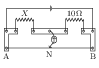A metre bridge is set-up as shown, to determine an unknown resistance $X$ using a standard $10\;\Omega$ resistor. The galvanometer shows null point when tapping-key is at 52 cm mark. The end corrections are 1 cm and 2 cm respectively for the ends A and B. The determined value of $X$ is,

1. $10.2\;\Omega$
2. $10.6\;\Omega$
3. $10.6\;\Omega$
4. $11.1\;\Omega$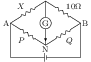Solution: Let N be the null point on the wire. Given $\mathrm{AN}=52\;\mathrm{cm}$ and $\mathrm{NB}=100-52=48\;\mathrm{cm}$. Let $A^\prime$ and $B^\prime$ represents two end points with end corrections i.e., $\mathrm{A^\prime N}=52+1=53\;\mathrm{cm}$ and $\mathrm{NB^\prime}=48+2=50\;\mathrm{cm}$. The resistance of branch $A^\prime N$ is $P=53\lambda$ and that of branch $N^\prime B$ is $Q=50\lambda$, where $\lambda$ is resistance per unit length (in ohm/cm). The Wheatstone bridge is balanced at null condition given by, \begin{align} &\frac{X}{10}=\frac{P}{Q}, & &\implies & &X=\frac{10P}{Q}=\frac{10(53\lambda)}{50\lambda}=10.6\;\Omega.\nonumber \end{align}

Problem (IIT JEE 2007): A resistance of $2\;\Omega$ is connected across one gap of a meter-bridge (the length of the wire is 100 cm) and an unknown resistance, greater than $2\;\Omega$, is connected across the other gap. When the resistances are interchanged, the balance point shifts by 20 cm. Neglecting any corrections, the unknown resistance is,

1. $3\;\Omega$
2. $4\;\Omega$
3. $5\;\Omega$
4. $6\;\Omega$

Solution: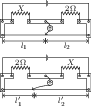Let $X$ be the unknown resistance. The null point is obtained when Wheatstone bridge is balanced i.e., $\frac{X}{2}=\frac{\rho l_1/A}{\rho l_2/A}=\frac{l_1}{l_2}$, where $l_1$ and $l_2$ are as shown in figure. Since $X > 2\;\Omega$, we get $l_1 > l_2$. Also, $l_1+l_2=100 \;\mathrm{cm}$. When resistances are interchanged, the null point shift by 20 cm. As $X > 2\;\Omega$, the null point will shift towards left i.e., $l_1^\prime=l_1-20$ and $l_2^\prime=l_2+20$. The balance condition gives $\frac{2}{X}=\frac{l_1^\prime}{l_2^\prime}=\frac{l_1-20}{l_2+20}$. Solve to get $X=3\;\Omega$.

Problem (IIT JEE 2004):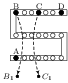For the post office box arrangement to determine the value of unknown resistance, the unknown resistance should be connected between,

1. B and C
2. C and D
3. A and D
4. $B_1$ and $C_1$

Solution: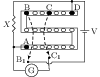The unknown resistance $X$ is connected between point A and D. Let resistance of branch BC be $P$, resistance of branch CD is $Q$, and resistance of rheostat branch AB be $R$. The Wheatstone bridge is balanced when $P/Q=R/X$ which gives $X=QR/P$.

Problem (IIT JEE 2003):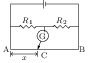In the shown arrangement of the experiment of the meter bridge if AC corresponding to null deflection of galvanometer is $x$, what should be its value if the radius of the wire AB is doubled?

1. $x$
2. $x/4$
3. $4x$
4. $2x$

If radius of the wire AB is doubled then its resistance ($R=\rho l/A$) becomes one fourth and current ($i=V/R$) becomes four times. However, the potential drop per unit length, $V/l$, remains same.

Problem (IIT JEE 2002):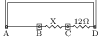A thin uniform wire AB of length 1 m, an unknown resistance $X$ and a resistance of $12\;\Omega$ are connected by thick conducting strips, as shown in figure. A battery and galvanometer (with a sliding jockey connected to it) are also available. Connections are to be made to measure the unknown resistance $X$ using the principle of Wheatstone bridge. Answer the following questions.

1. Are there positive and negative terminals on the galvanometer?
2. Copy the figure in your answer book and show the battery and the galvanometer (with jockey) connected at appropriate points.
3. After appropriate connections are made, it is found that no deflection takes place in the galvanometer when the sliding jockey touches the wire at a distance of 60 cm from A. Obtain the value of the resistance $X$.

Solution: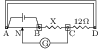The galvanometer does not have positive or negative terminals. The circuit diagram to measure unknown resistance $X$ is given in the figure. Let $R$ be total resistance of the potentiometer wire of length 100 cm and $N$ be the null point. The resistance of branch AN is $R_\text{AN}=60(R/100)=0.6R$ and that of branch BN is $R_\text{BN}=0.4R$. The balancing condition of Wheatstone bridge, $R_\text{AN}/R_\text{BN}=12/X$, gives $X=8\;\Omega$.